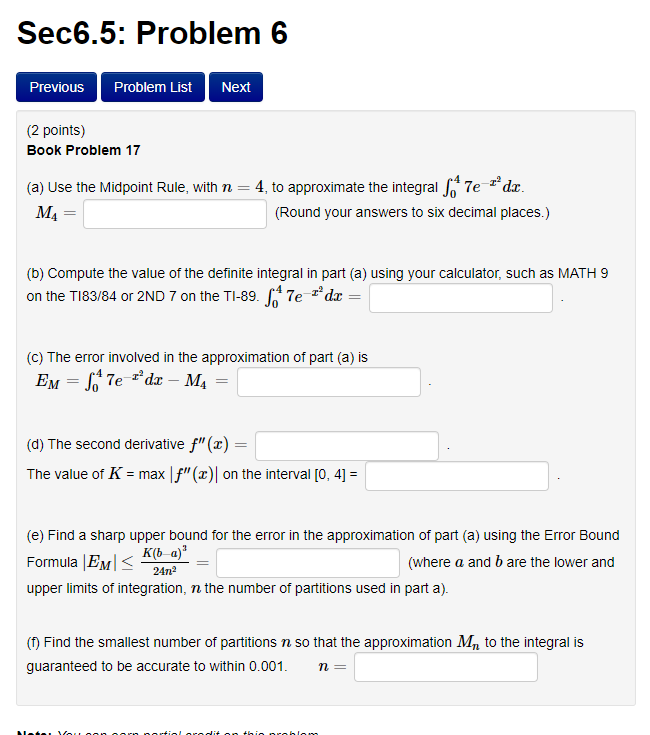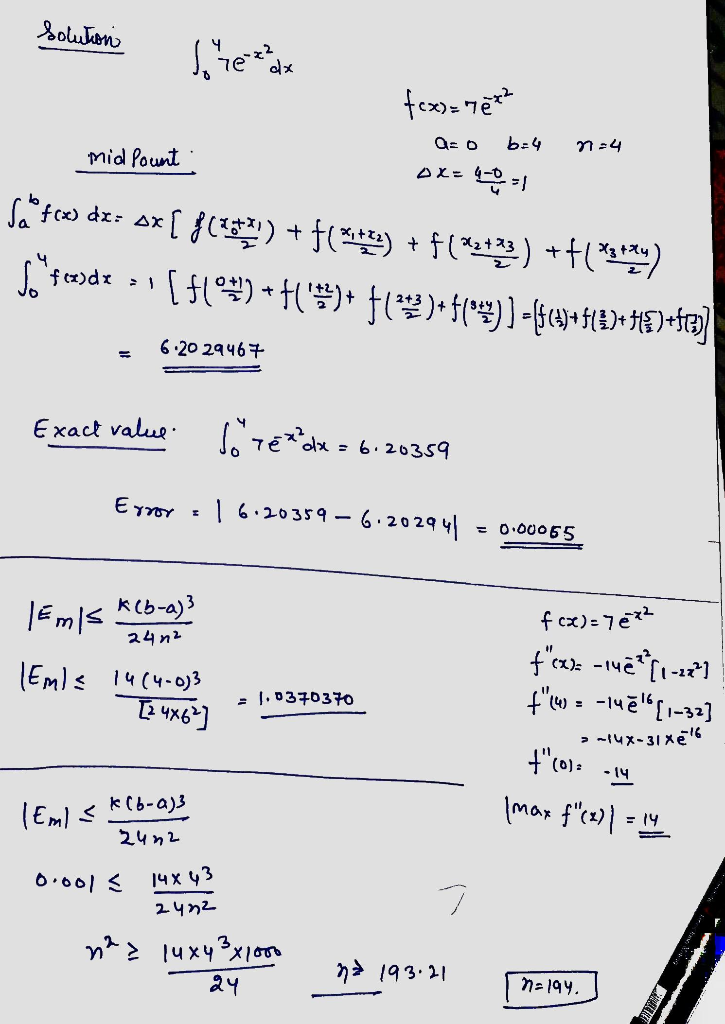# Sec6.5: Problem 6 Previous Problem List Next (2 points) Book Problem 17 4, to approximate the integral 7e dx (a) Use th...Sec6.5: Problem 6 Previous Problem List Next (2 points) Book Problem 17 4, to approximate the integral 7e dx (a) Use the Midpoint Rule, with n MA (Round your answers to six decimal places.) (b) Compute the value of the definite integral in part (a) using your calculator, such as MATH 9 on the TI83/84 or 2ND 7 on the TI-89. 7edx (c) The error involved in the approximation of part (a) is Ем — Те ах Ма (d) The second derivative f"(x) The value of K max | f" (x)| on the interval [0, 4] = (e) Find a sharp upper bound for the error in the approximation of part (a) using the Error Bound къ а)" Formula |EM upper limits of integration, n the number of partitions used in part a) (where a and b are the lower and 24n2 () Find the smallest number of partitions n so that the approximation M, to the integral is guaranteed to be accurate to within 0.001 n Aoe Vou oon ortiol rodito taio oroblon##### Add Answer of: Sec6.5: Problem 6 Previous Problem List Next (2 points) Book Problem 17 4, to approximate the integral 7e dx (a) Use th...
More Homework Help Questions Additional questions in this topic.